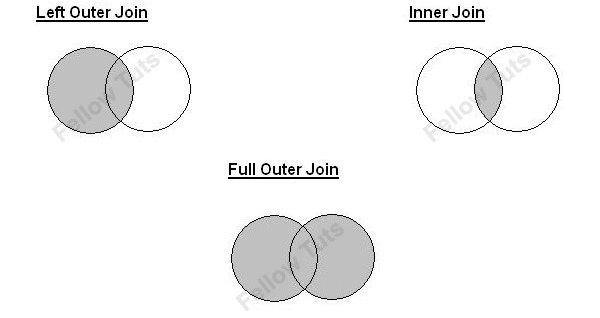# 3 easy MySQL examples – inner join, left outer & full outer join

Joins are fundamental concepts in SQL and they are prefer by professionals as they are faster then sub-queries.  These 3 easy MySQL examples below are a little effort to let visitors understand difference among Inner join, Left Outer join and Full outer join.

Assuming we’re joining on columns with no duplicates (which is a very common case), an inner join of P and Q gives the result of P intersect Q, i.e. the inner part of a venn diagram intersection and an outer join of P and Q gives the results of P union Q, i.e. the outer parts of a venn diagram union.### Easy Examples

Suppose we have two tables P and Q, with a single column each, and data as follows:

Here you can clearly sense that (1,2) are unique to P, (3,4) are common in both tables, and (5,6) are unique to Q.

### Inner Join

An inner join gives the rows they have in common means using either of the equivalent queries gives the intersection of the two tables (the two rows they have in common in our example).

### Left outer join

A left outer join will give all rows in P, plus any common rows in Q.

### Full outer join

All the rows in P and all the rows in Q, union of P and Q is given by full outer join. If something in P doesn’t have a corresponding datum in Q, then the Q portion is null, and vice versa.

Further to parse accurately, an outer join will give the results of A intersect B in addition to one of the following: all of P (left join), all of Q (right join) or all of P and all of Q (full join).

Also remember that Join does not guarantee an order, you would need to add an ORDER BY clause.

You Might Interested In